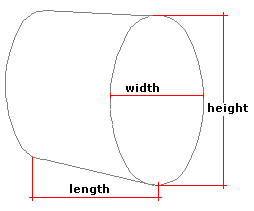Spike's Calculators

# Horizontal Elliptical Tank - On-Edge

Calculate the volume, capacity and surface area of a horizontal on-edge elliptical tank/container.

The tank here looks like a squashed circle, and for this type of tank/container, the height has to be larger than the width. This calculator uses inches for measurements.##### Note:
`The height measurement has to be larger than the width!`
##### Approximate Weight of Fuel or Water
```one US gallon of water = 8.34 pounds
one US gallon of diesel fuel = 7.1 pounds
one US gallon of gas = 6 pounds```

### Horizontal Elliptical Tank - On Edge

Height of the Tank in
Width of the Tank in
Length of the Tank in
Liquid Weight-Pounds per US Gallon lb/gal US

#### Results:

###### Tank Volume:
In Cubic Inches in³
In Cubic Feet ft³
In Cubic Metres
###### Tank Capacity:
In US Gallons gal US
In Imperial Gallons gal Imperial
In Litres L
###### Liquid Weight and Surface Area
Weight of Liquid lbs
Surface Area in Square Inches in²
In Square Feet ft²
In Square Millimetres mm²
In Square Metres

#### Calculator

1. enter the height of the tank in inches
2. the width of the tank in inches
3. the length of the tank in inches
4. the weight of the liquid in pounds per US gallon

#### Results

1. the volume of the tank in cubic inches
2. the volume in cubic feet
3. the volume in cubic metres
4. the tank capacity in US gallons
5. the capacity in Imperial gallons
6. the capacity in litres
7. full tank liquid weight
8. the surface area of the tank in square inches
9. the surface area in square feet
10. the surface area in square millimetres
11. the surface area in square metres

##### Formula
```volume= ΠHWL/4
where Π = 3.14159265
H is the height of the tank
W the width
L the length
```
##### Conversions
```one cubic inch (in³) = 0.000578703703703704 cubic feet (ft³)
one cubic inch (in³) = 0.000016387064 cubic metres (m³)
one cubic inch (in³) = 0.00432900432900432 gallons (gal) US
one cubic inch (in³) = 0.00360465014990905 gallons (gal) Imperial
one cubic inch (in³) = 0.016387064 litres (L)
```

### Ellipse-Horizontal Tank/Container (On-Edge)#### Filled On-Edge